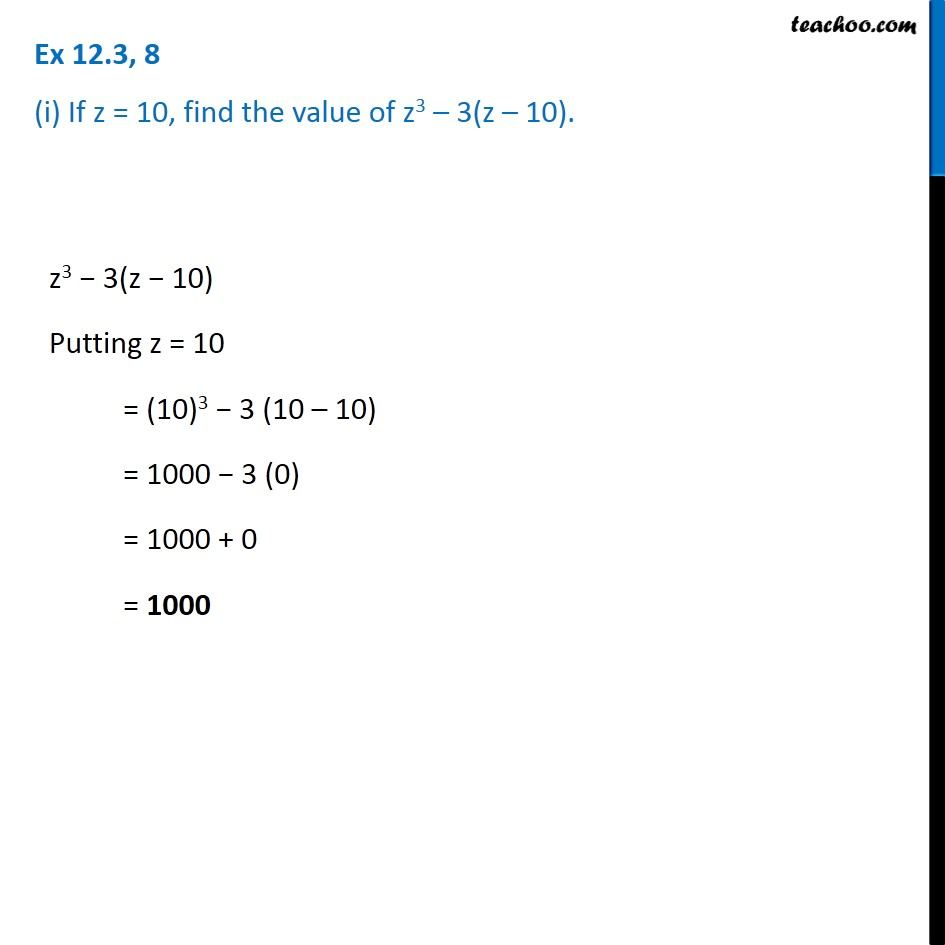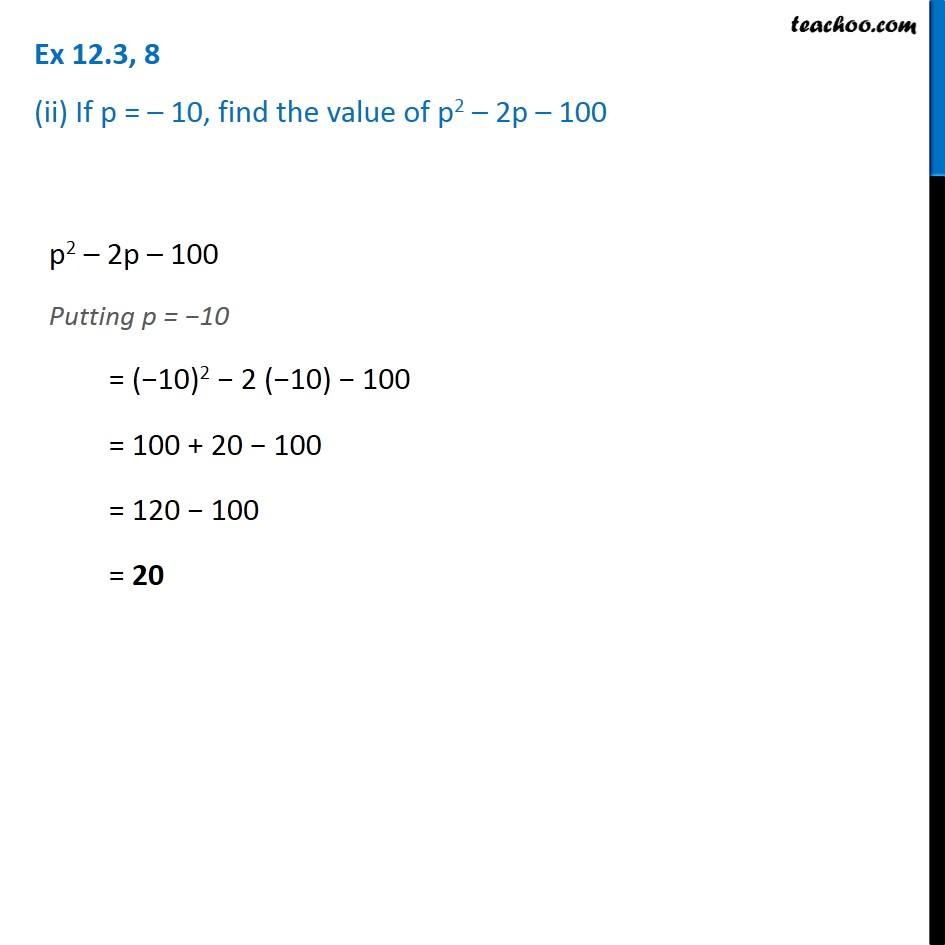1. Chapter 12 Class 7 Algebraic Expressions
2. Serial order wise
3. Ex 12.3

Transcript

Ex 12.3, 8 (i) If z = 10, find the value of z3 – 3(z – 10). z3 − 3(z − 10) Putting z = 10 = (10)3 − 3 (10 – 10) = 1000 − 3 (0) = 1000 + 0 = 1000 Ex 12.3, 8 (ii) If p = – 10, find the value of p2 – 2p – 100 p2 – 2p – 100 Putting p = −10 = (−10)2 − 2 (−10) − 100 = 100 + 20 − 100 = 120 − 100 = 20

Ex 12.3

About the AuthorDavneet Singh
Davneet Singh is a graduate from Indian Institute of Technology, Kanpur. He has been teaching from the past 10 years. He provides courses for Maths and Science at Teachoo.